# slLinearizer: Batch Linearization of Simulink Models

This week I am happy to welcome guest bloggers Charu Fadnavis and Erman Korkut to introduce the new slLinearizer interface from Simulink Control Design.linearize vs. slLinearizer

If you have used Simulink Control Design in the past, the first thing you might wonder is: Why a new interface to linearize Simulink models?

If you compare the linearize function with the slLinearizer interface, you will realize that many linear analysis tasks can be accomplished by both. The main advantage of the slLinearizer interface is that it makes it easier to obtain multiple transfer functions without recompiling the model, also known as Batch Linearization. To get more details on the differences between those two approaches, we recommend going through the documentation page Choosing Batch Linearization Tools.

The slLinearizer Interface

Let's go through a simple example illustrating the basic of the slLinearizer Interface. For that, we will use a very simple model: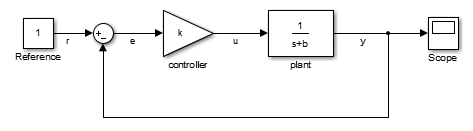The first step is to create a slLinearizer object for your model.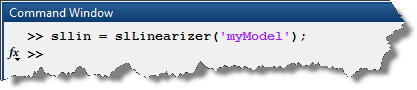Once the object is created, you want to add analysis points. One important difference when compared to the linearize workflow is that with the slLinearizer interface, the points that you specify are much more flexible. When adding points, you do not need to specify if they are input, output, open or closed loop, etc.

To add points, you can use block name and port number, or signal names. For this example, let's add multiple points.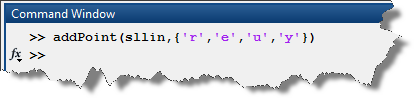We can now use any of the four get* methods to obtain transfer functions for the different points and loop openings: getIOTransfer, getLoopTransfer, getSensitivity, getCompSensitivity.

For example, if I want to get the transfer function between the reference r and the output y: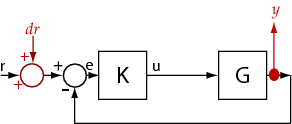I use getIOTransfer: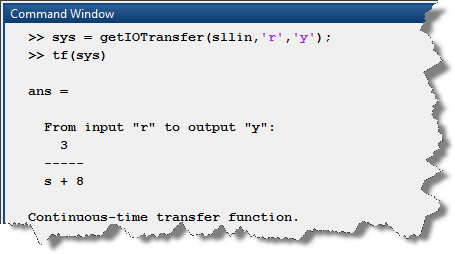If I want the sensitivity at the control signal u: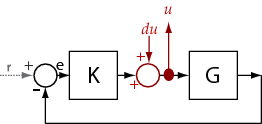I use getSensitivity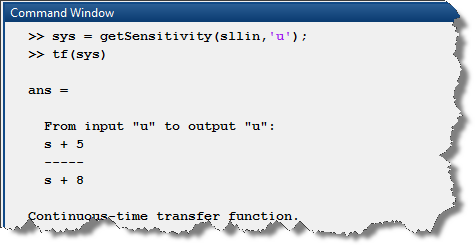The important thing to note, is that the model will be compiled only the first time one of the get* method is called.

Batch linearization

In addition to linearizing for multiple analysis points and loop opening without recompiling the model, the slLinearizer interface makes it easy to obtain multiple linearizations for a set of operating points or parameter values.

For the example above, let's say we want to obtain a series of transfer functions for different values of controller gain k, all you have to do is: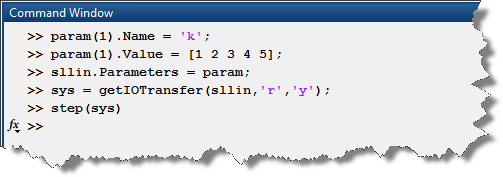and you obtain an array of transfer functions: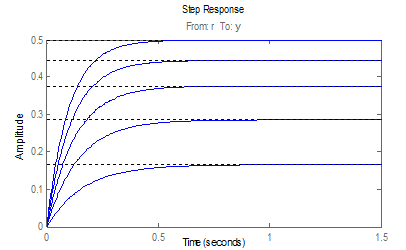If what you need to study is linearization at multiple operating points, it can also be done with the slLinearizer interface. For a detailed example of this workflow, see Vary Operating Points and Obtain Multiple Transfer Functions Using slLinearizer.